Mixture formation plays
21

Coefficient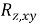can take values: 0<<1. When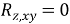between z and x and у there is no linear relationship with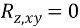= 1 value z is a linear functional dependence on x and y, as expressed, for example, the equation: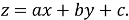Effect size x (or y) to replace r evaluate partial correlation coefficient.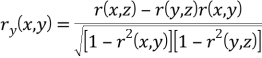Similarly, define r (z, y). Some correlation coefficients have the same properties as the linear correlation coefficients.

## 1.6 Dimensionless criteria of the atomization process

To achieve a fuller understanding of the atomisation process, a number of nondimensional numbers have been derived as follows:

• Weber number (We): expresses the ratio of dynamic forces of an ambient gas to the surface tension. It defines the effect of external factors on the drop development: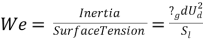where: ?g is the gas density, d the droplet diameter, ud the droplet relative velocity and s l the liquid surface tension.
• Reynolds number (Re): denotes the ratio of inertial force to viscous force: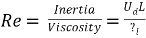where: L – nozzle length.
• Ohnesorge number (Oh): defines the ratio of internal viscous forces to surface tension forces: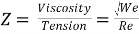.

These dimensionless numbers will be used in the structure of Diesel spray section to describe the break-up and the penetration length.